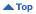Educational
HomeEducationalThe Sun and Solar ActivityGeneral InfoThe Equation of Time Friday, Aug 07 2020 12:47 UT

# Section Information

The Sun and Solar Activity

# The Equation of Time

The time on a sundial is not the same as the time you read from a watch. There are two reasons for this difference. One is due to our use of zonal times that are taken from fixed longitudes 15° apart. Australian Eastern Standard Time (AEST) is referred to a longitude of 150° east of the prime meridian, and this gives a time which is 10 hours ahead of Universal Time (excluding the effect of change in local time due to day light saving). Sydney (longitude 151.18°) is quite close to longitude 150°, and so when the Sun in Sydney reaches its highest point (e.g. on 10 July 2010) in the sky quite close to noon on a clock, in Melbourne the Sun would reach its highest point around 12.25 pm, Melbourne being about 6.2° west of Sydney in longitude.

There is a second effect which displaces the actual time of solar central meridian passage or CMP from the times listed above. This effect is called the "Equation of Time", and it is the time in minutes by which the time of CMP is displaced from mean solar noon (clock time).The equation of time results from:

• the 23° tilt of the Earth's rotational axis
• the fact that the Earth moves around the Sun in an elliptical orbit

When a planet moves in an elliptical orbit, it moves faster when it is at perihelion than when it is at aphelion. This appears to surface inhabitants as if the Sun moves around the Earth faster at perihelion (January) and slower at aphelion (July). The tilt of the Earth's axis makes the Sun appear to cross one's central meridian at different times than if the axis were perpendicular to the orbital plane. These two effects add to produce the equation of time as shown above.

The actual equation of time, which is mathematically defined as apparent solar time - mean solar time, varies slightly from year to year due to variations in the Earth's eccentricity and obliquity and in the time of the solstices and equinoxes. But, for a century either side of the year 2000 it may be approximated (to an accuracy of better than one percent) by the formula:

EoT(minutes) = 9.87 sin (2Bo) - 7.67 sin (Bo + 78.7)

where Bo = 360 (N-81) / 365 and N is the day of the year (eg N=1 on Jan 1 and N=33 on Feb 2)

The equation of time affects anything to do with the Sun, such as sunrise and sunset times as well as the CMP time.

Material prepared by Richard Thompson